GeeksforGeeks App
Open AppBrowser
Continue

## Related Articles

Functions in Excel are the predefined formulas that have their specific meaningful work. They make our task easy and quick like finding the sum, count, average, maximum value, and minimum values for a range of cells. For example, the SUM function will calculate the sum of the range passed to it as an argument.

Functions increase productivity while working in Excel.

### Basic Functions in Excel

We have data on Vegetables used in 1 week in a house, and we find the total cost of vegetables. Now, we’ll apply basic Excel Functions on the data.

• Sum(): It adds all the values in a range of cells.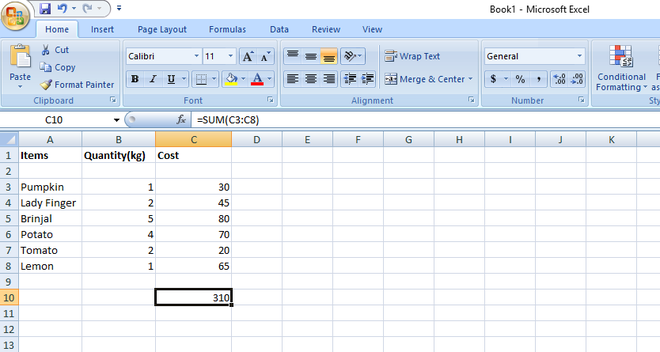=SUM(C3:C8) will find sum of values given in the range from C3 to C8.

• Max(): It will find the maximum value in the given range of values.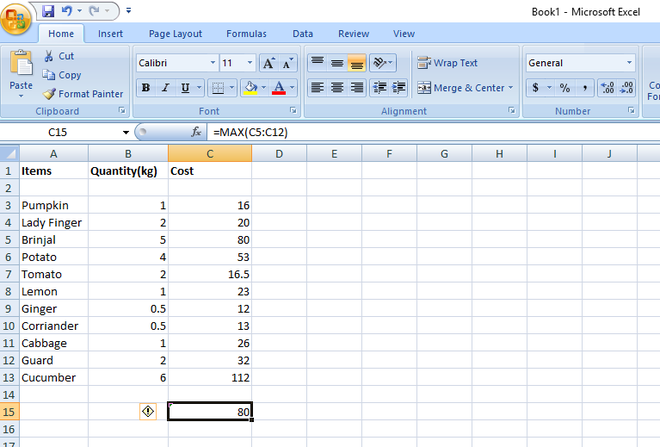Max value from C5 to C12 is 80.

• Min(): It will find the minimum value in the given range of values.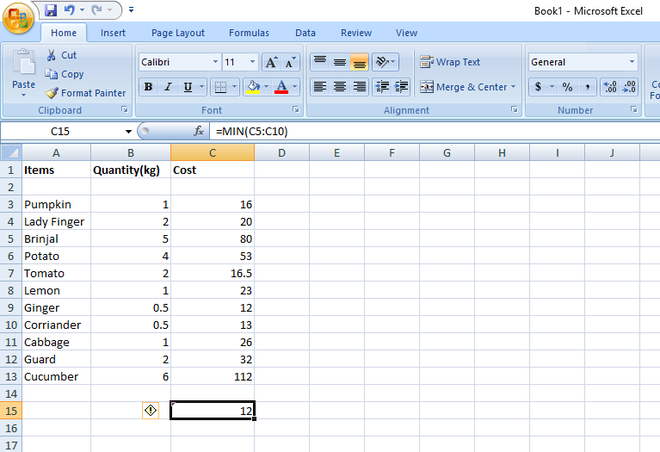MIn value from C5 to C10 is 12.

• Average(): It calculates the average value in a range of cells.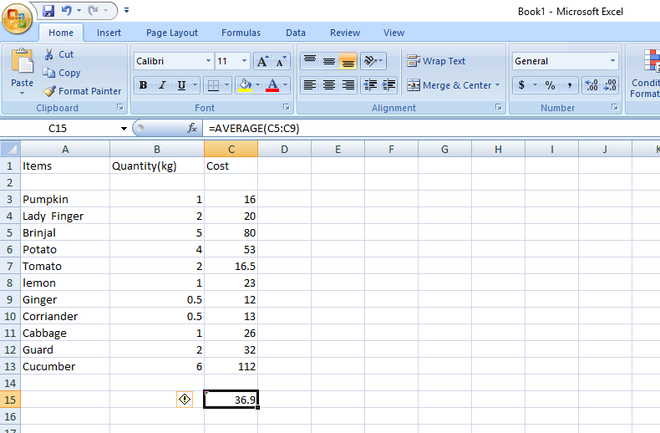• Count(): It will count the number of cells in a range of cells.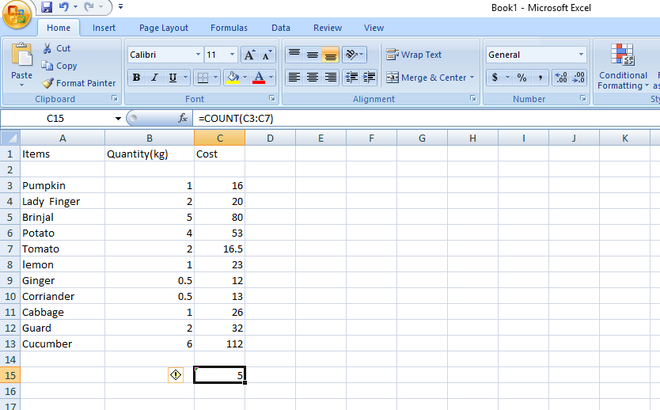• Len(): It will return the number of characters in a string text.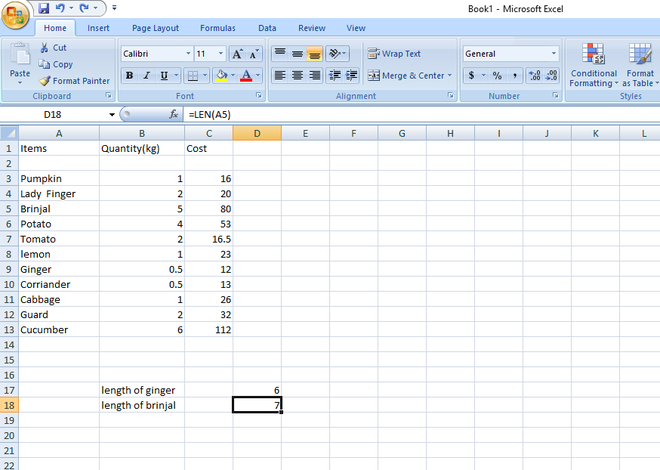• Sumif(): It Adds all the values in a range of cells that meet a specified condition. = SUMIF(range,criteria,[sum_range]).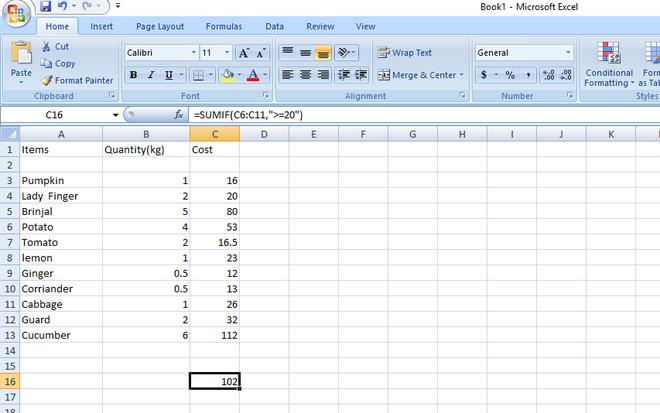• AverageIf():- It Calculates the average value in a range of cells that meet the specified criteria. =AVERAGEIF(range,criteria,[average_range]).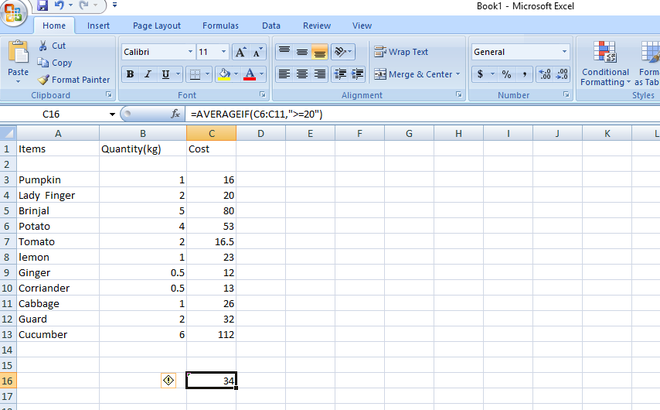My Personal Notes arrow_drop_up
Related Tutorials